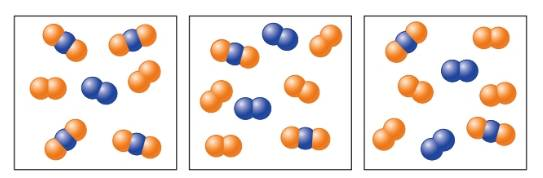Chapter 17, Problem 102AP### Introductory Chemistry: A Foundati...

9th Edition
Steven S. Zumdahl + 1 other
ISBN: 9781337399425

#### Solutions

Chapter
Section### Introductory Chemistry: A Foundati...

9th Edition
Steven S. Zumdahl + 1 other
ISBN: 9781337399425
Textbook Problem
211 views

# . Consider the following generic reaction: 2A 2 B ( g ) ⇌ 2A 2 ( g ) + B 2 ( g ) Some molecules of A2B are placed in a 1.0-L container. As time passes, several snapshots of the reaction mixture are taken as illustrated below.Which illustration is the first to represent an equilibrium mixture? Explain. How many molecules of A2B reacted initially?

Interpretation Introduction

Interpretation:

The illustration which represents an equilibrium mixture is to be predicted. The number of molecules of A2B that react initially is to be stated.

Concept Introduction:

In a chemical equation when the number of atoms of an element on the left-hand side is equal to the number of atoms of that element on the right-hand side, it is known as a balanced chemical equation. The balancing of a chemical equation is done to follow the law of conservation of mass.

The chemical equilibrium is the state in which the rates of both forward and backward reactions become equal. The concentration of both reactants and products do not change on reaching an equilibrium state.

The general chemical equation is shown below.

aA+bBcC+dD

The general equilibrium expression for a reaction is shown below.

K=CcDdAaBb.

Explanation

The given chemical equation is shown below.

2A2Bg2A2g+B2g

The first snapshot of the reaction mixture illustrates the presence of four A2B, two A2 and one B2 molecules. The second snapshot of the reaction mixture indicates the presence of two A2B, four A2 and two B2 molecules. The total number of molecules remains constant in the third snapshot.

Therefore, the second illustration is the first to represent an equilibrium mixture

### Still sussing out bartleby?

Check out a sample textbook solution.

See a sample solution

#### The Solution to Your Study Problems

Bartleby provides explanations to thousands of textbook problems written by our experts, many with advanced degrees!

Get Started